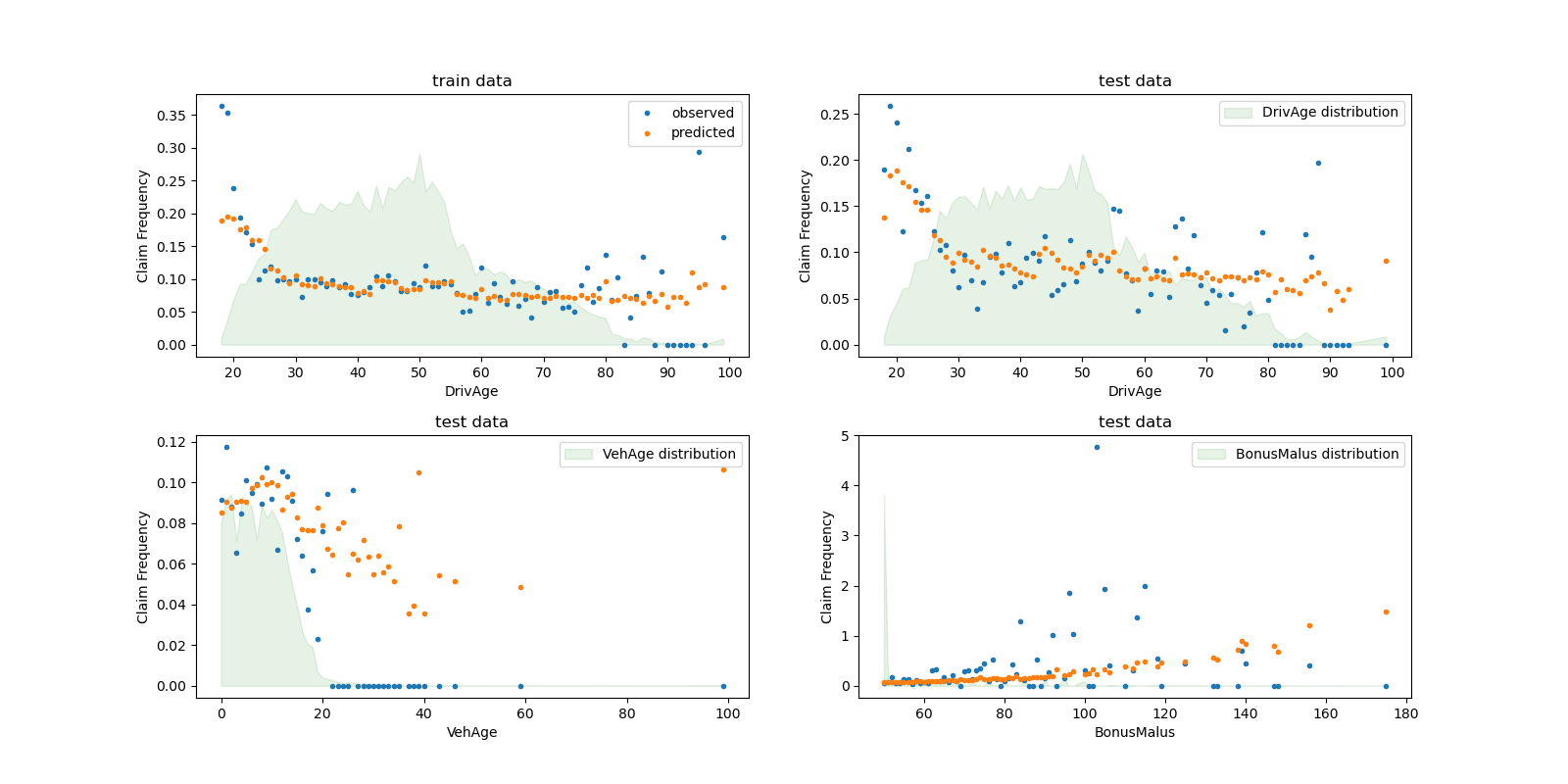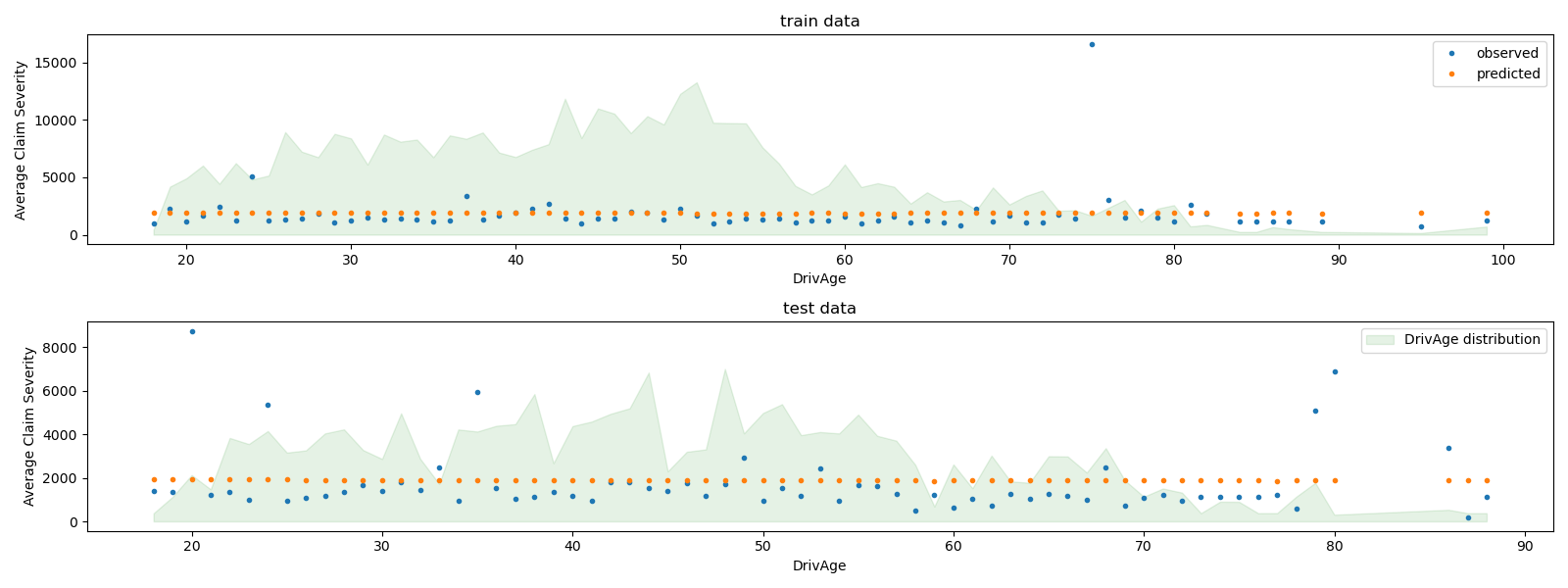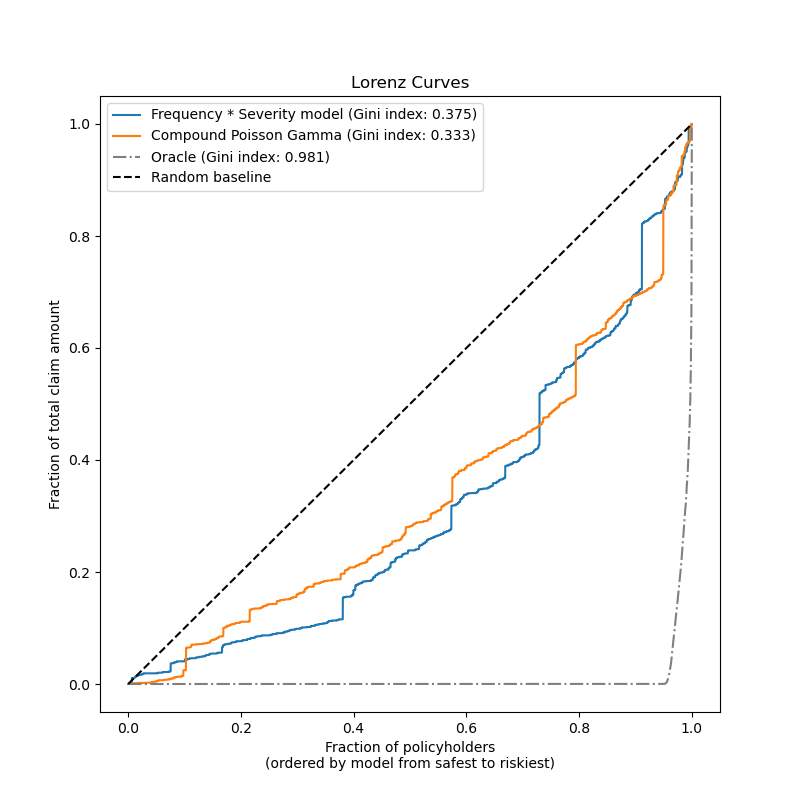# Tweedie regression on insurance claims¶

This example illustrates the use of Poisson, Gamma and Tweedie regression on the French Motor Third-Party Liability Claims dataset, and is inspired by an R tutorial 1.

In this dataset, each sample corresponds to an insurance policy, i.e. a contract within an insurance company and an individual (policyholder). Available features include driver age, vehicle age, vehicle power, etc.

A few definitions: a claim is the request made by a policyholder to the insurer to compensate for a loss covered by the insurance. The claim amount is the amount of money that the insurer must pay. The exposure is the duration of the insurance coverage of a given policy, in years.

Here our goal is to predict the expected value, i.e. the mean, of the total claim amount per exposure unit also referred to as the pure premium.

There are several possibilities to do that, two of which are:

1. Model the number of claims with a Poisson distribution, and the average claim amount per claim, also known as severity, as a Gamma distribution and multiply the predictions of both in order to get the total claim amount.

2. Model the total claim amount per exposure directly, typically with a Tweedie distribution of Tweedie power $$p \in (1, 2)$$.

In this example we will illustrate both approaches. We start by defining a few helper functions for loading the data and visualizing results.

1

A. Noll, R. Salzmann and M.V. Wuthrich, Case Study: French Motor Third-Party Liability Claims (November 8, 2018). doi:10.2139/ssrn.3164764

print(__doc__)

# Authors: Christian Lorentzen <lorentzen.ch@gmail.com>
#          Roman Yurchak <rth.yurchak@gmail.com>
#          Olivier Grisel <olivier.grisel@ensta.org>
from functools import partial

import numpy as np
import matplotlib.pyplot as plt
import pandas as pd

from sklearn.datasets import fetch_openml
from sklearn.compose import ColumnTransformer
from sklearn.linear_model import PoissonRegressor, GammaRegressor
from sklearn.linear_model import TweedieRegressor
from sklearn.metrics import mean_tweedie_deviance
from sklearn.model_selection import train_test_split
from sklearn.pipeline import make_pipeline
from sklearn.preprocessing import FunctionTransformer, OneHotEncoder
from sklearn.preprocessing import StandardScaler, KBinsDiscretizer

from sklearn.metrics import mean_absolute_error, mean_squared_error, auc

"""Fetch the French Motor Third-Party Liability Claims dataset.

Parameters
----------
n_samples: int, default=100000
number of samples to select (for faster run time). Full dataset has
678013 samples.
"""
# freMTPL2freq dataset from https://www.openml.org/d/41214
df_freq = fetch_openml(data_id=41214, as_frame=True)['data']
df_freq['IDpol'] = df_freq['IDpol'].astype(int)
df_freq.set_index('IDpol', inplace=True)

# freMTPL2sev dataset from https://www.openml.org/d/41215
df_sev = fetch_openml(data_id=41215, as_frame=True)['data']

# sum ClaimAmount over identical IDs
df_sev = df_sev.groupby('IDpol').sum()

df = df_freq.join(df_sev, how="left")
df["ClaimAmount"].fillna(0, inplace=True)

# unquote string fields
for column_name in df.columns[df.dtypes.values == object]:
df[column_name] = df[column_name].str.strip("'")
return df.iloc[:n_samples]

def plot_obs_pred(df, feature, weight, observed, predicted, y_label=None,
title=None, ax=None, fill_legend=False):
"""Plot observed and predicted - aggregated per feature level.

Parameters
----------
df : DataFrame
input data
feature: str
a column name of df for the feature to be plotted
weight : str
column name of df with the values of weights or exposure
observed : str
a column name of df with the observed target
predicted : DataFrame
a dataframe, with the same index as df, with the predicted target
fill_legend : bool, default=False
whether to show fill_between legend
"""
# aggregate observed and predicted variables by feature level
df_ = df.loc[:, [feature, weight]].copy()
df_["observed"] = df[observed] * df[weight]
df_["predicted"] = predicted * df[weight]
df_ = (
df_.groupby([feature])[[weight, "observed", "predicted"]]
.sum()
.assign(observed=lambda x: x["observed"] / x[weight])
.assign(predicted=lambda x: x["predicted"] / x[weight])
)

ax = df_.loc[:, ["observed", "predicted"]].plot(style=".", ax=ax)
y_max = df_.loc[:, ["observed", "predicted"]].values.max() * 0.8
p2 = ax.fill_between(
df_.index,
0,
y_max * df_[weight] / df_[weight].values.max(),
color="g",
alpha=0.1,
)
if fill_legend:
ax.legend([p2], ["{} distribution".format(feature)])
ax.set(
ylabel=y_label if y_label is not None else None,
title=title if title is not None else "Train: Observed vs Predicted",
)

def score_estimator(
estimator, X_train, X_test, df_train, df_test, target, weights,
tweedie_powers=None,
):
"""Evaluate an estimator on train and test sets with different metrics"""

metrics = [
("D² explained", None),   # Use default scorer if it exists
("mean abs. error", mean_absolute_error),
("mean squared error", mean_squared_error),
]
if tweedie_powers:
metrics += [(
"mean Tweedie dev p={:.4f}".format(power),
partial(mean_tweedie_deviance, power=power)
) for power in tweedie_powers]

res = []
for subset_label, X, df in [
("train", X_train, df_train),
("test", X_test, df_test),
]:
y, _weights = df[target], df[weights]
for score_label, metric in metrics:
if isinstance(estimator, tuple) and len(estimator) == 2:
# Score the model consisting of the product of frequency and
# severity models.
est_freq, est_sev = estimator
y_pred = est_freq.predict(X) * est_sev.predict(X)
else:
y_pred = estimator.predict(X)

if metric is None:
if not hasattr(estimator, "score"):
continue
score = estimator.score(X, y, sample_weight=_weights)
else:
score = metric(y, y_pred, sample_weight=_weights)

res.append(
{"subset": subset_label, "metric": score_label, "score": score}
)

res = (
pd.DataFrame(res)
.set_index(["metric", "subset"])
.score.unstack(-1)
.round(4)
.loc[:, ['train', 'test']]
)
return res


We construct the freMTPL2 dataset by joining the freMTPL2freq table, containing the number of claims (ClaimNb), with the freMTPL2sev table, containing the claim amount (ClaimAmount) for the same policy ids (IDpol).

df = load_mtpl2(n_samples=60000)

# Note: filter out claims with zero amount, as the severity model
# requires strictly positive target values.
df.loc[(df["ClaimAmount"] == 0) & (df["ClaimNb"] >= 1), "ClaimNb"] = 0

# Correct for unreasonable observations (that might be data error)
# and a few exceptionally large claim amounts
df["ClaimNb"] = df["ClaimNb"].clip(upper=4)
df["Exposure"] = df["Exposure"].clip(upper=1)
df["ClaimAmount"] = df["ClaimAmount"].clip(upper=200000)

log_scale_transformer = make_pipeline(
FunctionTransformer(func=np.log),
StandardScaler()
)

column_trans = ColumnTransformer(
[
("binned_numeric", KBinsDiscretizer(n_bins=10),
["VehAge", "DrivAge"]),
("onehot_categorical", OneHotEncoder(),
["VehBrand", "VehPower", "VehGas", "Region", "Area"]),
("passthrough_numeric", "passthrough",
["BonusMalus"]),
("log_scaled_numeric", log_scale_transformer,
["Density"]),
],
remainder="drop",
)
X = column_trans.fit_transform(df)

# Insurances companies are interested in modeling the Pure Premium, that is
# the expected total claim amount per unit of exposure for each policyholder
# in their portfolio:

# This can be indirectly approximated by a 2-step modeling: the product of the
# Frequency times the average claim amount per claim:
df["Frequency"] = df["ClaimNb"] / df["Exposure"]
df["AvgClaimAmount"] = df["ClaimAmount"] / np.fmax(df["ClaimNb"], 1)

with pd.option_context("display.max_columns", 15):


Out:

/home/circleci/project/sklearn/preprocessing/_discretization.py:220: UserWarning: Bins whose width are too small (i.e., <= 1e-8) in feature 0 are removed. Consider decreasing the number of bins.
warnings.warn('Bins whose width are too small (i.e., <= '
ClaimNb  Exposure Area  VehPower  VehAge  DrivAge  BonusMalus VehBrand  \
IDpol
139        1.0      0.75    F       7.0     1.0     61.0        50.0      B12
190        1.0      0.14    B      12.0     5.0     50.0        60.0      B12
414        1.0      0.14    E       4.0     0.0     36.0        85.0      B12
424        2.0      0.62    F      10.0     0.0     51.0       100.0      B12
463        1.0      0.31    A       5.0     0.0     45.0        50.0      B12

VehGas  Density Region  ClaimAmount   PurePremium  Frequency  \
IDpol
139    Regular  27000.0    R11       303.00    404.000000   1.333333
190     Diesel     56.0    R25      1981.84  14156.000000   7.142857
414    Regular   4792.0    R11      1456.55  10403.928571   7.142857
424    Regular  27000.0    R11     10834.00  17474.193548   3.225806
463    Regular     12.0    R73      3986.67  12860.225806   3.225806

AvgClaimAmount
IDpol
139            303.00
190           1981.84
414           1456.55
424           5417.00
463           3986.67


## Frequency model – Poisson distribution¶

The number of claims (ClaimNb) is a positive integer (0 included). Thus, this target can be modelled by a Poisson distribution. It is then assumed to be the number of discrete events occuring with a constant rate in a given time interval (Exposure, in units of years). Here we model the frequency y = ClaimNb / Exposure, which is still a (scaled) Poisson distribution, and use Exposure as sample_weight.

df_train, df_test, X_train, X_test = train_test_split(df, X, random_state=0)

# The parameters of the model are estimated by minimizing the Poisson deviance
# on the training set via a quasi-Newton solver: l-BFGS. Some of the features
# are collinear, we use a weak penalization to avoid numerical issues.
glm_freq = PoissonRegressor(alpha=1e-3, max_iter=400)
glm_freq.fit(X_train, df_train["Frequency"],
sample_weight=df_train["Exposure"])

scores = score_estimator(
glm_freq,
X_train,
X_test,
df_train,
df_test,
target="Frequency",
weights="Exposure",
)
print("Evaluation of PoissonRegressor on target Frequency")
print(scores)


Out:

Evaluation of PoissonRegressor on target Frequency
subset               train    test
metric
D² explained        0.0591  0.0580
mean abs. error     0.1706  0.1661
mean squared error  0.3041  0.3043


We can visually compare observed and predicted values, aggregated by the drivers age (DrivAge), vehicle age (VehAge) and the insurance bonus/malus (BonusMalus).

fig, ax = plt.subplots(ncols=2, nrows=2, figsize=(16, 8))

plot_obs_pred(
df=df_train,
feature="DrivAge",
weight="Exposure",
observed="Frequency",
predicted=glm_freq.predict(X_train),
y_label="Claim Frequency",
title="train data",
ax=ax[0, 0],
)

plot_obs_pred(
df=df_test,
feature="DrivAge",
weight="Exposure",
observed="Frequency",
predicted=glm_freq.predict(X_test),
y_label="Claim Frequency",
title="test data",
ax=ax[0, 1],
fill_legend=True
)

plot_obs_pred(
df=df_test,
feature="VehAge",
weight="Exposure",
observed="Frequency",
predicted=glm_freq.predict(X_test),
y_label="Claim Frequency",
title="test data",
ax=ax[1, 0],
fill_legend=True
)

plot_obs_pred(
df=df_test,
feature="BonusMalus",
weight="Exposure",
observed="Frequency",
predicted=glm_freq.predict(X_test),
y_label="Claim Frequency",
title="test data",
ax=ax[1, 1],
fill_legend=True
)According to the observed data, the frequency of accidents is higher for drivers younger than 30 years old, and is positively correlated with the BonusMalus variable. Our model is able to mostly correctly model this behaviour.

## Severity Model - Gamma distribution¶

The mean claim amount or severity (AvgClaimAmount) can be empirically shown to follow approximately a Gamma distribution. We fit a GLM model for the severity with the same features as the frequency model.

Note:

• We filter out ClaimAmount == 0 as the Gamma distribution has support on $$(0, \infty)$$, not $$[0, \infty)$$.

• We use ClaimNb as sample_weight to account for policies that contain more than one claim.

mask_train = df_train["ClaimAmount"] > 0

glm_sev = GammaRegressor(alpha=10., max_iter=10000)

glm_sev.fit(
)

scores = score_estimator(
glm_sev,
target="AvgClaimAmount",
weights="ClaimNb",
)
print("Evaluation of GammaRegressor on target AvgClaimAmount")
print(scores)


Out:

Evaluation of GammaRegressor on target AvgClaimAmount
subset                     train          test
metric
D² explained        4.300000e-03 -1.380000e-02
mean abs. error     1.699197e+03  2.027923e+03
mean squared error  4.548147e+07  6.094863e+07


Here, the scores for the test data call for caution as they are significantly worse than for the training data indicating an overfit despite the strong regularization.

Note that the resulting model is the average claim amount per claim. As such, it is conditional on having at least one claim, and cannot be used to predict the average claim amount per policy in general.

print("Mean AvgClaim Amount per policy:              %.2f "
% df_train["AvgClaimAmount"].mean())
print("Mean AvgClaim Amount | NbClaim > 0:           %.2f"
% df_train["AvgClaimAmount"][df_train["AvgClaimAmount"] > 0].mean())
print("Predicted Mean AvgClaim Amount | NbClaim > 0: %.2f"
% glm_sev.predict(X_train).mean())


Out:

Mean AvgClaim Amount per policy:              97.89
Mean AvgClaim Amount | NbClaim > 0:           1899.60
Predicted Mean AvgClaim Amount | NbClaim > 0: 1884.40


We can visually compare observed and predicted values, aggregated for the drivers age (DrivAge).

fig, ax = plt.subplots(ncols=1, nrows=2, figsize=(16, 6))

plot_obs_pred(
feature="DrivAge",
weight="Exposure",
observed="AvgClaimAmount",
y_label="Average Claim Severity",
title="train data",
ax=ax,
)

plot_obs_pred(
feature="DrivAge",
weight="Exposure",
observed="AvgClaimAmount",
y_label="Average Claim Severity",
title="test data",
ax=ax,
fill_legend=True
)
plt.tight_layout()Overall, the drivers age (DrivAge) has a weak impact on the claim severity, both in observed and predicted data.

## Pure Premium Modeling via a Product Model vs single TweedieRegressor¶

As mentioned in the introduction, the total claim amount per unit of exposure can be modeled as the product of the prediction of the frequency model by the prediction of the severity model.

Alternatively, one can directly model the total loss with a unique Compound Poisson Gamma generalized linear model (with a log link function). This model is a special case of the Tweedie GLM with a “power” parameter $$p \in (1, 2)$$. Here, we fix apriori the power parameter of the Tweedie model to some arbitrary value (1.9) in the valid range. Ideally one would select this value via grid-search by minimizing the negative log-likelihood of the Tweedie model, but unfortunately the current implementation does not allow for this (yet).

We will compare the performance of both approaches. To quantify the performance of both models, one can compute the mean deviance of the train and test data assuming a Compound Poisson-Gamma distribution of the total claim amount. This is equivalent to a Tweedie distribution with a power parameter between 1 and 2.

The sklearn.metrics.mean_tweedie_deviance depends on a power parameter. As we do not know the true value of the power parameter, we here compute the mean deviances for a grid of possible values, and compare the models side by side, i.e. we compare them at identical values of power. Ideally, we hope that one model will be consistently better than the other, regardless of power.

glm_pure_premium = TweedieRegressor(power=1.9, alpha=.1, max_iter=10000)
sample_weight=df_train["Exposure"])

tweedie_powers = [1.5, 1.7, 1.8, 1.9, 1.99, 1.999, 1.9999]

scores_product_model = score_estimator(
(glm_freq, glm_sev),
X_train,
X_test,
df_train,
df_test,
weights="Exposure",
tweedie_powers=tweedie_powers,
)

X_train,
X_test,
df_train,
df_test,
weights="Exposure",
tweedie_powers=tweedie_powers
)

axis=1, sort=True,
keys=('Product Model', 'TweedieRegressor'))
print("Evaluation of the Product Model and the Tweedie Regressor "
with pd.option_context('display.expand_frame_repr', False):
print(scores)


Out:

Evaluation of the Product Model and the Tweedie Regressor on target PurePremium
Product Model               TweedieRegressor
subset                            train          test            train          test
metric
D² explained                        NaN           NaN     2.550000e-02  2.480000e-02
mean Tweedie dev p=1.5000  8.217340e+01  8.646200e+01     7.960780e+01  8.618680e+01
mean Tweedie dev p=1.7000  3.833470e+01  3.922390e+01     3.737380e+01  3.917450e+01
mean Tweedie dev p=1.8000  3.106740e+01  3.149880e+01     3.047890e+01  3.148120e+01
mean Tweedie dev p=1.9000  3.396140e+01  3.421260e+01     3.360060e+01  3.420820e+01
mean Tweedie dev p=1.9900  1.989235e+02  1.996459e+02     1.986911e+02  1.996461e+02
mean Tweedie dev p=1.9990  1.886428e+03  1.892752e+03     1.886206e+03  1.892753e+03
mean Tweedie dev p=1.9999  1.876452e+04  1.882692e+04     1.876430e+04  1.882692e+04
mean abs. error            3.246724e+02  3.469663e+02     3.202456e+02  3.397020e+02
mean squared error         1.469185e+08  3.325937e+07     1.469327e+08  3.325470e+07


In this example, both modeling approaches yield comparable performance metrics. For implementation reasons, the percentage of explained variance $$D^2$$ is not available for the product model.

We can additionally validate these models by comparing observed and predicted total claim amount over the test and train subsets. We see that, on average, both model tend to underestimate the total claim (but this behavior depends on the amount of regularization).

res = []
for subset_label, X, df in [
("train", X_train, df_train),
("test", X_test, df_test),
]:
exposure = df["Exposure"].values
res.append(
{
"subset": subset_label,
"observed": df["ClaimAmount"].values.sum(),
"predicted, frequency*severity model": np.sum(
exposure * glm_freq.predict(X) * glm_sev.predict(X)
),
"predicted, tweedie, power=%.2f"
}
)

print(pd.DataFrame(res).set_index("subset").T)


Out:

subset                                      train          test
observed                             4.577616e+06  1.725665e+06
predicted, frequency*severity model  4.565402e+06  1.495568e+06
predicted, tweedie, power=1.90       4.451547e+06  1.431960e+06


Finally, we can compare the two models using a plot of cumulated claims: for each model, the policyholders are ranked from safest to riskiest and the fraction of observed total cumulated claims is plotted on the y axis. This plot is often called the ordered Lorenz curve of the model.

The Gini coefficient (based on the area under the curve) can be used as a model selection metric to quantify the ability of the model to rank policyholders. Note that this metric does not reflect the ability of the models to make accurate predictions in terms of absolute value of total claim amounts but only in terms of relative amounts as a ranking metric.

Both models are able to rank policyholders by risky-ness significantly better than chance although they are also both far from perfect due to the natural difficulty of the prediction problem from few features.

Note that the Gini index only characterize the ranking performance of the model but not its calibration: any monotonic transformation of the predictions leaves the Gini index of the model unchanged.

Finally one should highlight that the Compound Poisson Gamma model that is directly fit on the pure premium is operationally simpler to develop and maintain as it consists in a single scikit-learn estimator instead of a pair of models, each with its own set of hyperparameters.

def lorenz_curve(y_true, y_pred, exposure):
y_true, y_pred = np.asarray(y_true), np.asarray(y_pred)
exposure = np.asarray(exposure)

# order samples by increasing predicted risk:
ranking = np.argsort(y_pred)
ranked_exposure = exposure[ranking]
cumulated_claim_amount /= cumulated_claim_amount[-1]
cumulated_samples = np.linspace(0, 1, len(cumulated_claim_amount))
return cumulated_samples, cumulated_claim_amount

fig, ax = plt.subplots(figsize=(8, 8))

y_pred_product = glm_freq.predict(X_test) * glm_sev.predict(X_test)

for label, y_pred in [("Frequency * Severity model", y_pred_product),
("Compound Poisson Gamma", y_pred_total)]:
ordered_samples, cum_claims = lorenz_curve(
gini = 1 - 2 * auc(ordered_samples, cum_claims)
label += " (Gini index: {:.3f})".format(gini)
ax.plot(ordered_samples, cum_claims, linestyle="-", label=label)

# Oracle model: y_pred == y_test
ordered_samples, cum_claims = lorenz_curve(
gini = 1 - 2 * auc(ordered_samples, cum_claims)
label = "Oracle (Gini index: {:.3f})".format(gini)
ax.plot(ordered_samples, cum_claims, linestyle="-.", color="gray",
label=label)

# Random baseline
ax.plot([0, 1], [0, 1], linestyle="--", color="black",
label="Random baseline")
ax.set(
title="Lorenz Curves",
xlabel=('Fraction of policyholders\n'
'(ordered by model from safest to riskiest)'),
ylabel='Fraction of total claim amount'
)
ax.legend(loc="upper left")
plt.plot()Out:

[]


Total running time of the script: ( 0 minutes 28.892 seconds)

Gallery generated by Sphinx-Gallery